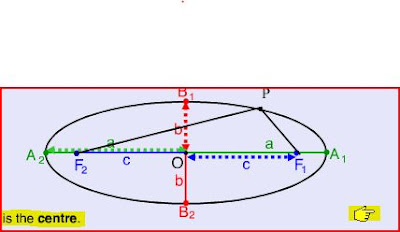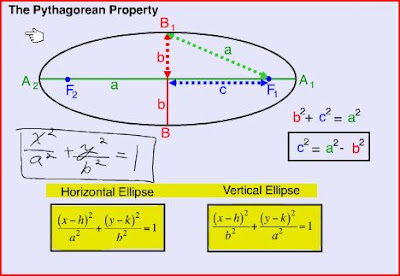Thursday, May 21, 2009

May21st/09

Today we started on the ellipses part of the unit.
if you take the cone again and cut it across at an angle it creates an ellipse.Reference Jessi.
The word ellipses comes from Greek: (ἔλλειψις ellipse) Means a "falling short". ...
By definition:
The locus of points for which the sum of the distances from each point to two fixed points is equal.
Then Mr.K showed us the tip of drawing ellipses: use 2 pin and a elastic. (it's in the slides #4)In this diagram there is few point to remember about ellipses:
• O is the centre
• the line A1 to A2 is the length of the major axis, the length is 2a.(Major axis is the longer axis of the ellipses)
• PF1& Pf2 are the focal radii of the ellipses
• OA1=OA2 are the length of the semimajor axis with length a. (Semimajor means half of the Major axis)
• B1B2 is the length of the Minor axis. it's length is 2b.(Minor axis is the shorter axis of the ellipse)
• OB1=OB2 is the length of the Semiminor axis with length b. (Semiminor axis means half of the minor axis).
• F1 and F2 are called the foci, they are c units from then centre. ( foci because it's more than one)
• A1 and A2 are the vertices of the ellipses.
• B1 and B2 are the endpoints of the minor axis.
Difference between vertical and horizontal ellipses:
Vertical-major axis is going up and down
-minor axis is going left to right
horizontal-major axis is going left to right
-minor axis is going up and downRemember it's not always a^2+b^2=c^2 in this case it's a^2+c^2=b^2
In an ellipses a endpoint of the minor axis connect with a focus point Will form a right triangle.the 2 highlighted formula are ellipses for both horizontal and vertical.
In the diagram shown how the Pythagorean works for the ellipses.In this problem we used the formula fist we find the vertex: which is (3,-1) same as circle.
then 25 is a^2 and 9 is b^2 so the distance of semimajor is 5 and semiminor is 3.
from there we fin c using a^2+c^2=b^2. therefore c is 4 the foci are 4 units left and right of the centre point.

And basically that all we have done today, and be careful don't forget to do ur home work!!!!

Next scribe is misuiz